# Test: Tabulation

## 18 Questions MCQ Test General Intelligence and Reasoning for SSC CHSL | Test: Tabulation

Description
Attempt Test: Tabulation | 18 questions in 20 minutes | Mock test for SSC preparation | Free important questions MCQ to study General Intelligence and Reasoning for SSC CHSL for SSC Exam | Download free PDF with solutions
QUESTION: 1

### Direction: The table given below shows the production of maize by 5 different states as a percentage of total production. Each state produces only maize and rice. There are three types of rice - R1, R2 and R3. The table also shows the R1 type of rice produced as a percentage of total rice production and the ratio of R2 and R3 type of rice. Total production by each state is 625000.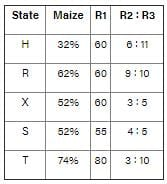A = Average of the R3 type of rice produced by state H, R, S and X together. B = Difference between the R2 type of rice produced by state T and R1 type of rice produced by state R. What is the value of K/F? (where K = Average of the total production of R1 type of rice by all the states and F = Total production of R2 type of rice by all the states.)

Solution:

F = Total production of R2 type of rice by all the states.
⇒ F = (6/17 × 0.4 × 0.68 + 9/19 × 0.4 × 0.38 + 3/8 × 0.4 × 0.48 + 4/9 × 0.45 × 0.48 + 3/13 × 0.2 × 0.26) × 625000
⇒ (0.096 + 0.072 + 0.072 + 0.096 + 0.012) × 625000
⇒ 0.348 × 625000
⇒ 217500
K = Average of the total production of R1 type of rice by all the states.
⇒ Total production of R1 type of rice by all the states = (0.6 × 0.68 + 0.6 × 0.38 + 0.6 × 0.48 + 0.55 × 0.48 + 0.8 × 0.26) × 625000
⇒ (0.408 + 0.228 + 0.288 + 0.264 + 0.208) × 625000
⇒ (1.396) × 625000
⇒ 872500
⇒ K = 872500/5 = 174500
∴ K/F = 174500/217500 = 0.802

QUESTION: 2

### Direction: The table given below shows the production of maize by 5 different states as a percentage of total production. Each state produces only maize and rice. There are three types of rice - R1, R2 and R3. The table also shows the R1 type of rice produced as a percentage of total rice production and the ratio of R2 and R3 type of rice. Total production by each state is 625000.A = Average of the R3 type of rice produced by state H, R, S and X together. B = Difference between the R2 type of rice produced by state T and R1 type of rice produced by state R. What is the value of B - A?

Solution:

⇒ R3 type of rice produced by state H = 11/17 × 0.4 × 0.68 × 625000 = 110000
⇒ R3 type of rice produced by state R = 10/19 × 0.4 × 0.38 × 625000 = 50000
⇒ R3 type of rice produced by state S = 5/9 × 0.45 × 0.48 × 625000 = 75000
⇒ R3 type of rice produced by state X = 5/8 × 0.4 × 0.48 × 625000 = 75000
⇒ A = Average of the R3 type of rice produced by state H, R, S and X together
⇒ A = (110000 + 50000 + 75000 + 75000) /4 = 77500
⇒ R2 type of rice produced by state T = 3/13 × 0.2 × 0.26 × 625000 = 7500
⇒ R1 type of rice produced by state R = 0.6 × 0.38 × 625000 = 142500
⇒ B = Difference between the R2 type of rice produced by state T and R1 type of rice produced by state R.
⇒ B = 142500 - 7500 = 135000
∴ B - A = 135000 - 77500 = 57500

QUESTION: 3

### Direction: The table given below shows the production of maize by 5 different states as a percentage of total production. Each state produces only maize and rice. There are three types of rice - R1, R2 and R3. The table also shows the R1 type of rice produced as a percentage of total rice production and the ratio of R2 and R3 type of rice. Total production by each state is 625000.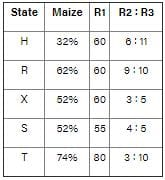A = Average of the R3 type of rice produced by state H, R, S and X together. B = Difference between the R2 type of rice produced by state T and R1 type of rice produced by state R. Production of R3 type of rice by state X is what percentage of production of R1 type of rice by state S?

Solution:

Quantity of rice produced by state X = 0.48 × 625000 = 300000
⇒ (R2 and R3) type of rice produced by state X = 300000 × 0.40 = 120000
⇒ R3 type rice produced in state X = 5/8 × 120000 = 75000
And
⇒ Quantity of rice produced by state S = 625000 × 0.48 = 300000
⇒ R1 type rice produced in state S = 300000 × 0.55 = 165000
∴ Required percentage = (75000/165000) × 100 = 45.45%
A = Average of the R3 type of rice produced by state H, R, S and X together.
B = Difference between the R2 type of rice produced by state T and R1 type of rice produced by state R.

QUESTION: 4

Direction: The table given below shows the production of maize by 5 different states as a percentage of total production. Each state produces only maize and rice. There are three types of rice - R1, R2 and R3. The table also shows the R1 type of rice produced as a percentage of total rice production and the ratio of R2 and R3 type of rice. Total production by each state is 625000.A = Average of the R3 type of rice produced by state H, R, S and X together.
B = Difference between the R2 type of rice produced by state T and R1 type of rice produced by state R.

What is the sum of the total production of maize by state X and T and total production of R2 type of Rice by state S and R?

Solution:

Total production of maize by state X and T = 625000 × (0.52 + 0.74) = 787500
Now
⇒ Quantity of rice produced by state S = 625000 × 0.48 = 300000
⇒ (R2 and R3) type of rice produced by state S = 300000 × 0.45 = 135000
⇒ R2 type of rice produced in state S = 4/9 × 135000 = 60000
And
⇒ Quantity of rice produced by state R = 625000 × 0.38 = 237500
⇒ (R2 and R3) type of rice produced by state R = 237500 × 0.4 = 95000
⇒ R2 type of rice produced in state R = 9/19 × 95000 = 45000
⇒ Total production of R2 type of Rice by state S and R = 60000 + 45000 = 105000
∴ Required sum = 787500 + 105000 = 892500

QUESTION: 5

Direction: The table given below shows the production of maize by 5 different states as a percentage of total production. Each state produces only maize and rice. There are three types of rice - R1, R2 and R3. The table also shows the R1 type of rice produced as a percentage of total rice production and the ratio of R2 and R3 type of rice. Total production by each state is 625000.A = Average of the R3 type of rice produced by state H, R, S and X together.
B = Difference between the R2 type of rice produced by state T and R1 type of rice produced by state R.

What is the difference between the R1 type of rice produced by state X and the R2 type of rice produced by state H?

Solution:

Rice produced by state X = 48% and that of by state H = 68%
⇒ Quantity of rice produced by state X = 0.48 × 625000 = 300000
⇒ R1 type of rice produced by state X = 300000 × 0.6 = 180000
Now
⇒ Quantity of rice produced by state H = 0.68 × 625000 = 425000
⇒ R1 type of rice produced by state H = 425000 × 0.6 = 255000
⇒ Remaining quantity of rice = 425000 - 255000 = 170000
⇒ R2 type of rice produced by state H = 6/17 × 170000 = 60000
∴ Required difference = 180000 - 60000 = 120000

QUESTION: 6

Direction: The table given below shows the income and expenditure (in Rs) of two companies A and B form 2012 to 2016.
Also, Profit = Income - Expenditure
and Profit% = [(Income - Expenditure)/Expenditure] × 100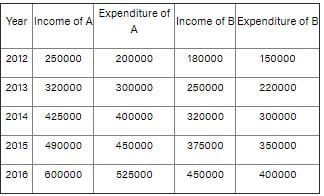What is the ratio of profit of A in year 2013 and profit of B in year 2016 respectively?

Solution:

Profit of A in year 2013 = 3,20,000 - 3,00,000 = Rs. 20,000
Profit of B in year 2016 = 4,50,000 - 4,00,000 = Rs. 50,000
Ratio = 20000/50000 = 2 : 5

QUESTION: 7

Direction: The table given below shows the income and expenditure (in Rs) of two companies A and B form 2012 to 2016.
Also, Profit = Income - Expenditure
and Profit% = [(Income - Expenditure)/Expenditure] × 100What is the average yearly profit (in Rs) of A for the given years?

Solution:

Average income of A = (250000 + 320000 + 425000 + 490000 + 600000)/5 = Rs. 4,17,000
Average expenditure of A = (200000 + 300000 + 400000 + 450000 + 525000)/5= Rs. 3,75,000
Average profit of A = 417000 - 375000 = Rs. 42,000

QUESTION: 8

Direction: The table given below shows the income and expenditure (in Rs) of two companies A and B form 2012 to 2016.
Also, Profit = Income - Expenditure
and Profit% = [(Income - Expenditure)/Expenditure] × 100For which year the profit percentage of B is the highest?

Solution:

Profit percentage of B for 2012 = (180000 - 150000)/150000 × 100 = 20%
Profit percentage of B for 2013 = (250000 - 220000)/220000 × 100 = 13.64%
Profit percentage of B for 2014 = (320000 - 300000)/300000 × 100 = 6.67%
Profit percentage of B for 2015 = (375000 - 350000)/350000 × 100 = 7.14%
Profit percentage of B for 2016 = (450000 - 400000)/400000 × 100 = 12.5%
The profit percentage of B for 2012 is the highest

QUESTION: 9

Direction: The table given below shows the income and expenditure (in Rs) of two companies A and B form 2012 to 2016.
Also, Profit = Income - Expenditure
and Profit% = [(Income - Expenditure)/Expenditure] × 100If the income and expenditure of A increases by same rate in 2017 over 2016 as they did in 2016 over 2015, then what is its profit percentage (approximate) in 2017?

Solution:

Income of A in 2017 = 600000 × (600000/490000) = Rs. 7,34,693.88
Expenditure of A in 2017 = 525000 × (525000/450000) = Rs. 6,12,500
Profit percent = (734693.88 - 612500)612500 × 100 = 20%

QUESTION: 10

Direction: The table given below shows the income and expenditure (in Rs) of two companies A and B form 2012 to 2016.
Also, Profit = Income - Expenditure
and Profit% = [(Income - Expenditure)/Expenditure] × 100The number of students passing in all streams was maximum in the year.

Solution:

From the given data,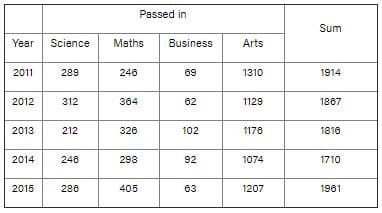As we can see from the given table, the number of students passing in all streams was maximum in the year 2015.

QUESTION: 11

Directions : Study the following table which shows the number of candidates appeared and passed indifferent streams in a School and answer the questions given below it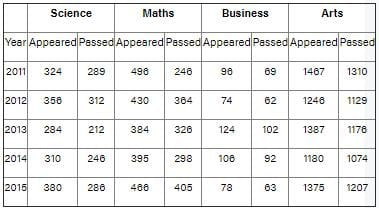The number of students appearing in all streams was minimum in the year

Solution:

From the given data,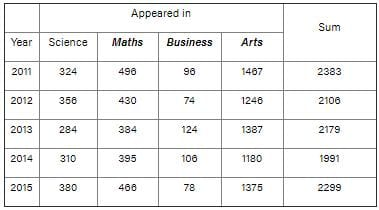As we can see from the given table, the number of students appearing in all streams was minimum in the year 2014

QUESTION: 12

Directions : Study the following table which shows the number of candidates appeared and passed indifferent streams in a School and answer the questions given below it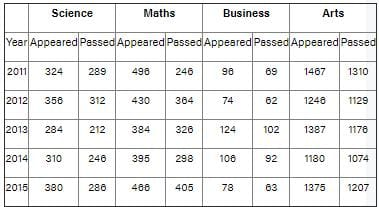Approximately what per cent of total students appearing in 2014, appeared in Arts stream?

Solution:

Total students appearing in 2014 = 310 + 395 + 106 + 1180 = 1991
Students appearing in Arts stream in 2014 = 1180
Per cent of total students appearing in 2014, appeared in Arts stream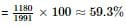QUESTION: 13

Directions : Study the following table which shows the number of candidates appeared and passed indifferent streams in a School and answer the questions given below it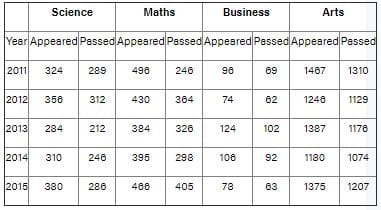Approximately what percent of students appearing in Maths, passed in 2013?

Solution:

Number of students appearing in Maths = 384
Number of students passed in 2013 = 326
Percentage of students appearing in Maths, passed in 2013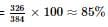QUESTION: 14

Directions: Study the following table and answer the questions: Population of a locality from 1988 to 1992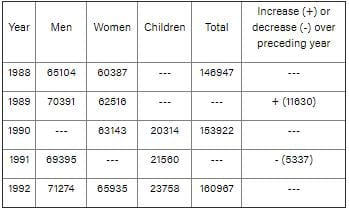What is the ratio of children and men in 1992?

Solution:

The number of children in 1992 is = 23758
The number of men in 1992 is = 71274
Therefore, ratio is 1:3

QUESTION: 15

Directions: Study the following table and answer the questions: Population of a locality from 1988 to 1992Number of women in 1991 is:

Solution:

∵ Total population in 1990 = 153922
∵ Decrease I population in 1991 over the preceding year = 5337
∵ Total population in 1991 = 153922 – 5337 = 148585
∴ Number of women in 1991 = 148585 – (69395 + 21560) = 57630

QUESTION: 16

Directions: Study the following table and answer the questions: Population of a locality from 1988 to 1992Number of children in 1989 is:

Solution:

∵ Total population in 1988 = 146947
∵ Increase in population in 1989 over preceding year = 11630
∵ Total population in 1989 = 146947 + 11630 = 158577
∴ Number of children in 1989 = 158577 – (70391 + 62516) = 25670

QUESTION: 17

Directions: Study the following table and answer the questions: Population of a locality from 1988 to 1992What is the population of men in 1990?

Solution:

∵ Total population in 1990 = 153922
∴ Number of men in 1990 = 153922 – (63143 + 20314) = 70465

QUESTION: 18

Directions: Study the following table and answer the questions: Population of a locality from 1988 to 1992The number of children in 1988 is

Solution:

∵ Total population in 1988 = 146947
∴ Number of children in 1988 = 146947 – (65104 + 60387) = 21456Use Code STAYHOME200 and get INR 200 additional OFF Use Coupon Code